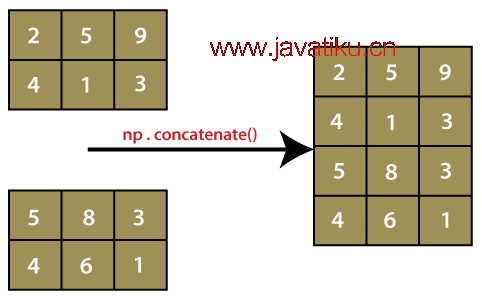# NumPy教程-使用numpy.concatenate()在Python中连接数组numpy.concatenate() 函数是NumPy包中的函数。该函数主要用于将NumPy数组组合在一起。此函数基本上用于在指定的轴上连接两个或多个具有相同形状的数组。有以下几点需要注意：

1. NumPy的concatenate()不像传统的数据库连接那样，它更像是将NumPy数组堆叠在一起。
2. 此函数可以在垂直和水平方向上操作。这意味着我们可以将数组水平或垂直连接在一起。concatenate() 函数通常写作 np.concatenate()，但我们也可以写成 numpy.concatenate()。这取决于导入numpy包的方式，即使用 `import numpy as np` 还是 `import numpy`

## 语法

``numpy.concatenate((a1, a2, ...), axis)``

1) (a1, a2, ...)

2) axis：int（可选）

## 示例 1：numpy.concatenate()

``````import numpy as np
x = np.array([[1,2],[3,4]])
y = np.array([[12,30]])
z = np.concatenate((x,y))
z ``````

• 我们使用别名np导入numpy。
• 我们使用np.array()函数创建一个数组'x'。
• 然后，我们使用相同的np.array()函数创建另一个数组'y'。
• 我们声明变量'z'并将其赋值为np.concatenate()函数的返回值。
• 我们在函数中传递数组'x'和'y'。
• 最后，我们尝试打印'z'的值。

``````array([[ 1,  2],
[ 3,  4],
[12, 30]])``````

## 示例 2：numpy.concatenate() 使用 axis=0

``````import numpy as np
x = np.array([[1,2],[3,4]])
y = np.array([[12,30]])
z = np.concatenate((x,y), axis=0)
z ``````

``````array([[ 1,  2],
[ 3,  4],
[12, 30]])``````

## 示例 3：numpy.concatenate() 使用 axis=1

``````import numpy as np
x = np.array([[1,2],[3,4]])
y = np.array([[12,30]])
z = np.concatenate((x,y.T), axis=1)
z ``````

``````array([[ 1,  2, 12],
[ 3,  4, 30]])``````

## 示例 4：numpy.concatenate() 使用 axis=None

``````import numpy as np
x = np.array([[1,2],[3,4]])
y = np.array([[12,30]])
z = np.concatenate((x,y), axis=None)
z ``````

``array([ 1,  2,  3,  4, 12, 30])``

## 示例 5：np.ma.concatenate()

``````import numpy as np
x = np.ma.arange(3)
y = np.arange(3,6)
x
y
z1 = np.concatenate([x,y])
z2 = np.ma.concatenate([x,y])
z1
z2 ``````

• 我们使用别名np导入numpy。
• 我们使用np.ma.arange()函数创建一个数组'x'。
• 然后，我们使用相同的np.ma.arange()函数创建另一个数组'y'。
• 我们声明变量'z1'并将其赋值为np.concatenate()函数的返回值。
• 我们声明变量'z2'并将其赋值为np.ma.concatenate()函数的返回值。
• 最后，我们尝试打印'z1'和'z2'的值。

``````masked_array(data=[0, --, 2],